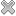# Courses / Module

Toggle Print

##PROBABILITY AND STATISTICS

Module code: EE304FZ
Credits: 5
Semester: 1
Department: INTERNATIONAL ENGINEERING COLLEGE
International:Overview

Probability Theory
� Introduction to Probability Theory and Forms of Data Presentation - Contingency Tables
� Review of Basic Set Theory
� Definition of Events in terms of sets
� Complementary and Mutually Exclusive Events.
� Interpretation of Probability in terms of relative frequencies.
� Axioms of Probability Theory
� Conditional Probability
� Bayes Theorem and Law of Total Probability
� Concept of Independent Events
� Network Problems and Determination of Reliability of Networks
� Counting Techniques and Application to Sample Spaces with large numbers of sample points
� Discrete Random Variables and the Probability mass function
� Special Discrete Probability Distributions � Binomial, Poisson Distribution
� Application of Poisson distribution to engineering problems - Queuing Theory
� Expected Value and Variance, Chebychev's Inequality
� Continuous Random Variables (Probability Density Function and Cumulative Distribution Function)
� Properties of a probability density, expected value and variance.
� The Gaussian (Normal) distribution and its properties and importance in application.
� The exponential distribution and its relation to the Poisson process and queuing theory
� The Gamma distribution and the Weibull Distribution and their application to modelling times to failure

Statistics
� Introduction to Statistics � Inference and Estimation of Parameters.
� The central limit theorem.
� Large/Small Sample confidence interval estimates for the population mean and the T-distribution.
� Large Sample confidence interval estimates for a population proportion.
� Introduction to hypothesis testing and the idea behind the process.
� Hypothesis testing on a population mean (large and small samples).
� Hypothesis testing on a population proportion (large sample).
� Categorical Data: Chi-squared goodness of fit test. Chi-squared independence test.
� Simple Linear Regression. Correlation/Causation. Prediction Intervals. Hypothesis testing.
� Discussion of relation of studied material to simple engineering experiment design.Learning OutcomesTeaching & Learning methodsAssessmentRepeat optionsTimetable